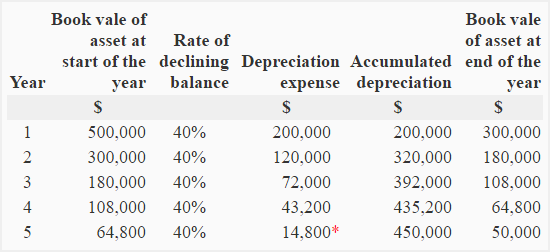Declining balance method of depreciation

The declining balance method is a widely used form of accelerated depreciation in which some percentage of straight line depreciation rate is used. A usual practice is to apply a 200% or 150% of the straight line rate to calculate depreciation expense for the period. The depreciation rate that is determined in this way is known as declining balance rate or accelerated depreciation rateFor example, if straight line depreciation rate is 10% and the company uses a 200% of the straight line depreciation rate, the accelerated depreciation rate to be used in declining balance method would be 20% (10% × 2).

Unlike other depreciation methods, the salvage value is not deducted from the cost of the asset under this method. The accelerated depreciation rate is applied to the book value (undepreciated cost) of the asset at the beginning of the period. The continuous charge of depreciation reduces book value of the asset year by year. When book value of the asset is reduced to its salvage, no more depreciation is provided.

Double declining balance method:

The double declining balance method is simply a declining balance method in which double (200%) of the straight line depreciation rate is used. Also discussed in the first paragraph of the article.

Formula:

The first step in declining balance method is to calculate a straight line depreciation rate, that is calculated using the following formula:

Straight-line depreciation rate = 1/Useful life of the asset

After calculating straight line rate, the accelerated depreciation rate is calculated to be used in the declining balance method. It is calculated using the following formula:

Accelerated depreciation rate = straight-line depreciation rate × Specific percentage

Finally, the depreciation expense is calculated using the following formula:

Depreciation expense = Remaining book value × Accelerated depreciation rate

Example:

The Farma company provides the following information:

Cost of the equipment: \$500,000

Salvage value: \$50,000

Useful life: 5 years

Required: Prepare a depreciation schedule using double declining balance method.

Solution:

Step 1 – Straight-line depreciation rate:

1/5 = 0.2 or 20%

Step 2 – Declining balance rate (accelerated depreciation rate):

20% × 2 = 40%

Step 3 – calculation of depreciation expense and preparation of schedule:* The book value of the equipment at the beginning of year 5 is \$64,800 whose 40% is \$2,5920. If this depreciation is used, the book value of the equipment at the end of year 5 will be \$38,880 (\$64,800 – \$25,920) that is less than the salvage value. As stated earlier, the asset is depreciated only to its salvage value under declining balance method. Therefore, the depreciation in year 5 has been provided as follows:

= Book value at the beginning of the year – Salvage value

= \$64,800 – \$50,000

= \$14,800

Show your love for us by sharing our contents.

3 Comments on Declining balance method of depreciation

1.Ramin

thank,s a bundle it was so defecult to me but thw way that u explained was so easy

2.TESFAYE DEJENE

Its good ideas for understanding.

3.Chinenye